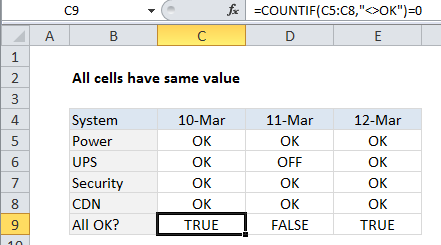## Excel Office

Excel How Tos, Tutorials, Tips & Tricks, Shortcuts

# Check multiple cells have same value in Excel

To confirm that a range of cells all have the same value, you can use a formula based on the COUNTIF function.

See illustration below:

## Formula

`=COUNTIF(range,"<>value")=0`## Explanation

In the example shown, the formula in C9 is:

`=COUNTIF(C5:C8,"<>ok")=0`

Note: this formula is not case-sensitive, you can find a case-sensitive formula here.

Also See:   Count Unique Values in Excel

### How this formula works

This formula relies on the standard behavior of the COUNTIF function. The range is C5:C8, the criteria is provided as not equals OK:

`=COUNTIF(C5:C8,"<>ok")`

The COUNTIF then returns a count of any cells that do not contain “OK” which is compared to zero. If the count is zero, the formula returns TRUE. If the count is anything but zero, the formula returns FALSE.

Also See:   Count cells that contain positive numbers in Excel

### Ignore empty cells

To ignore empty cells, you can use a more generic version of the formula:

`=COUNTIF(range,value)=COUNTA(range)`

This formula generates a count of all matching values, and compares that count to a count of all non-empty cells.